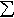#Interactive Real Analysis

Next | Previous | Glossary | Map

## 7.4. Lebesgue Integral

### Example 7.4.2(a): Simple Functions

A step function is a function s(x) such that s(x) = cj for xj-1 < x < xj and the { xj } form a partition of [a, b]. Upper, Lower, and Riemann sums are examples of step functions. What is the difference, if any, between step functions and simple functions.
Suppose s(x) = cj for xj-1 < x < xj and the { xj } form a partition of [a, b]. Define functions Xj(x) such that Xj = 1 if xj-1 < x < xj and 0 otherwise. Then each Xj is a characteristic function of the interval [xj-1, xj] and the sum
S(x) =cj Xj(x)
is a simple function because (sub)intervals are measurable. But S(x) = s(x), so that every step function is also a simple function.

But not every simple function is a step function. Take, for example, the set Q of rational numbers inside [0, 1] and A = [2, 3]. Then the function

S(x) = XQ(x) + XA(x)
is a simple function but not a step function.

Therefore, simple functions are more general than step functions.

Next | Previous | Glossary | Map